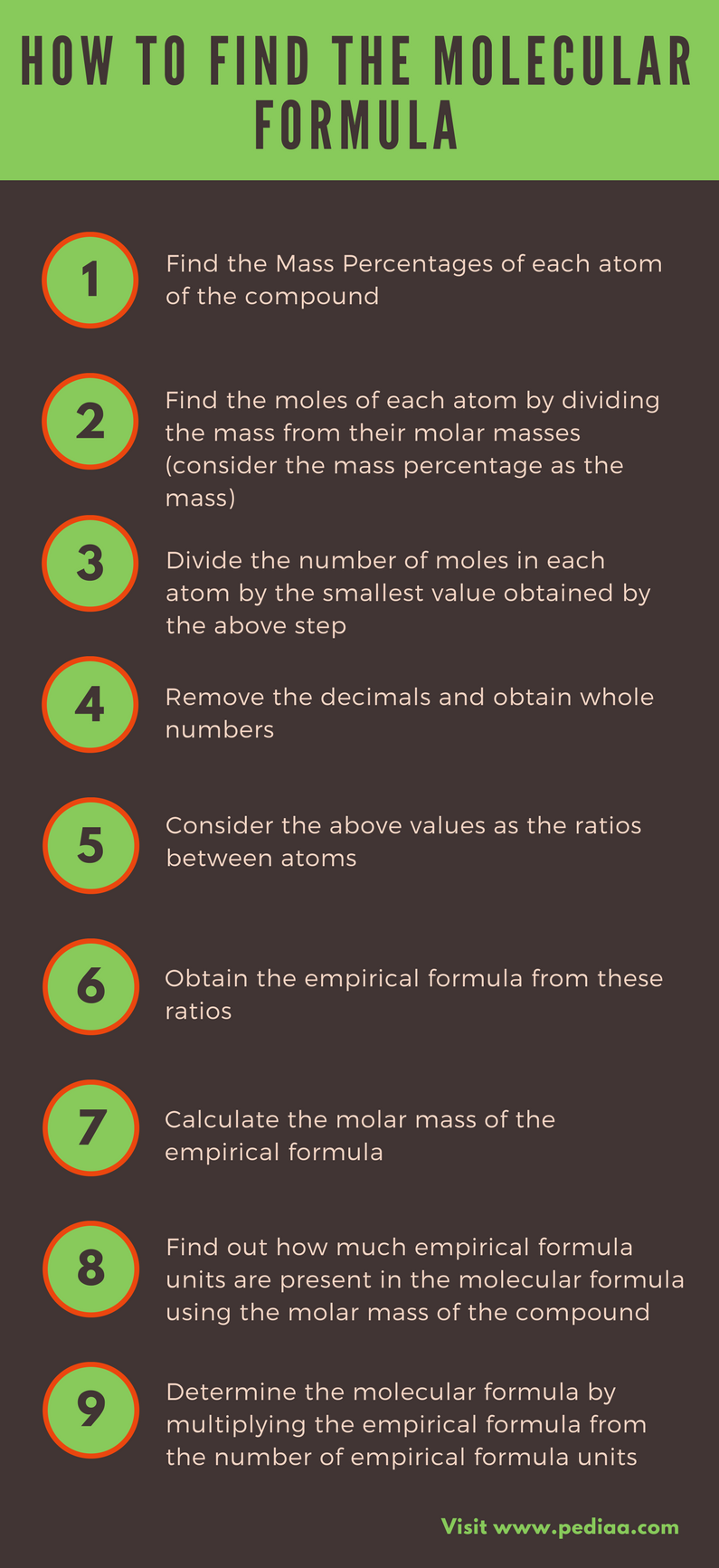# How to Find the Molecular Formula

The molecular formula is a way of expressing the atoms and their amounts present in a compound. The molecular formula of a compound is represented by elemental symbols and their ratios. Thus, it is very important to know how to find the molecular formula of a compound in order to know the elements present in a compound and their amounts.

Key Terms: Atomic Mass, Carbon, Empirical Formula, Hydrogen, Molar Mass, Molecular Formula, Oxygen

## Key Areas Covered

1. What is Molecular Formula
– Definition and Explanation
2. How to Find the Molecular Formula
– Using the Empirical Formula
3. Why is it Important to Know the Molecular Formula
– Applications of Molecular Formula## What is Molecular Formula

The molecular formula is the chemical formula of a compound that gives information about the types and number of atoms the compound is made of. But it does not give information about the structural arrangement of those atoms. It also does not give information about the types and number of bonds present in between those atoms.

## How to Find the Molecular Formula

The molecular formula of a compound can be found with the use of empirical formula. The empirical formula is the chemical formula which gives the ratio between the atoms present in the compound. It does not give the exact number of each atom present.

Before finding the molecular formula, one should find out the empirical formula from the mass percentages of each atom present in the compound. Let’s consider the following example.

Our compound is composed of 36 % of carbon (C), 58% of oxygen (O) and the rest is hydrogen (H). The molar mass of the compound is given as 60 g/mol.

• First, assume that we have 100 g of the compound.
• The percentage of H present is = 100 – (36+58) = 6%
• Therefore, the amount of C present is 36 g

H present is 6 g

O present is 58 g

• Then we can find the moles of each atom by dividing the mass from their molar masses.

C = 36 g/ 12 gmol-1  = 3 mol

H = 6 g/ 1 gmol-1      = 6 mol

O = 58 g/ 16 gmol-1 = 3.625 mol

• Divide the number of moles in each atom by the smallest number among them.

C = 3/3

H = 6/3

O = 3.625/3

• Remove the decimals and obtain whole numbers.

C = 1

H = 2

O = 1.208    (round this off to 1)

• Consider the above values as the ratios between atoms.
• Then the empirical formula can be obtained by these ratios.

The empirical formula is CH2O.

• The molar mass was given as 60 g/mol. Then, we should find out how much CH2O units are present there. For that, first, calculate the molar mass of empirical formula and then divide the given molar mass value from the calculated value.

Molar mass of CH2O   = (1 x 12) + (2 x 1) + (1 x 16)

= 12 + 2 + 16

= 30

Therefore the number of CH2O units present in the compound are

= 60/30

= 2

Therefore the compound should be (CH2O) x 2

= C2H4O2Figure 01: A suggestion for C2H4O2 molecule

## Why is it Important to Know the Molecular Formula?

The molecular formula gives information about the types of atoms present in a compound and their ratios. Knowing the molecular formula is useful in classification of compounds. And also it represents the molar mass of a compound. The easiest way to find the molar mass is by adding the atomic masses of each atom in a compound. Therefore, the molecular formula is the key to find out the molar mass.

### Conclusion

The way of finding the molecular formula of a compound is explained in this article. To find the molecular formula, the mass percentages of each atom in that compound should be known.

##### Image Courtesy:

1. “Essigsäure – Acetic acid” By NEUROtiker – Own work, Public Domain) via Commons Wikimedia

##### References:

1.Helmenstine, Anne Marie. “How to Determine the Empirical and Molecular Formulas of a Compound.” ThoughtCo. N.p., n.d. Web. Available here. 22 June 2017.
2. “Molecular Formula 2.” Chem.Ed. N.p., n.d. Web. Available here. 22 June 2017.